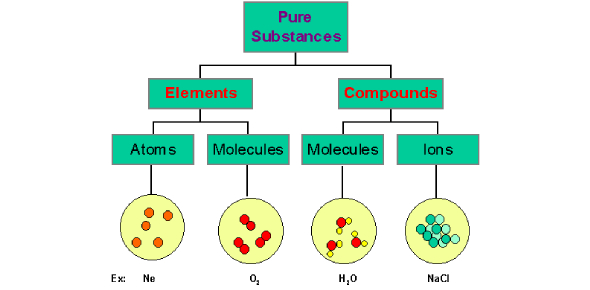# Quiz On Elements And Compounds Of Atom!

10 Questions | Attempts: 10380SettingsAn atom is a primary piece of matter made up of protons, neutrons, and electrons called subatomic particles. The atom's center is known as the Nucleus, which carries the negative charge around which electrons flow. This quiz has been developed to test your knowledge about atomic numbers, protons, nuclear mass, and neutrons. Let's take the quiz.

• 1.
If lithium has an atomic number of 3 and a mass number of 7, the correct number of protons, neutrons, and electrons, respectively, is:
• A.

3,4,3

• B.

4,3,3

• C.

3,3,4

• D.

4,4,3

• 2.
If Magnesium has an atomic number of 12 and a mass number of 24, the correct number of protons, neutrons, and electrons, respectively, is:
• A.

8,10,12

• B.

12,12,12

• C.

12,24,12

• D.

24,12,12

• 3.
If Zinc has an atomic number of 30 and a mass number of 65, the correct number of protons, neutrons, and electrons, respectively, is:
• A.

30,35,35

• B.

30,35,30

• C.

35,30,35

• D.

95,65,30

• 4.
If Bromine has an atomic number of 35 and has 45 neutrons, what is its mass number?
• A.

35

• B.

45

• C.

10

• D.

80

• 5.
If Aluminum has an atomic number of 13 and has 14 neutrons, what is its mass number?
• A.

1

• B.

27

• C.

13

• D.

14

• 6.
If Uranium's atomic number is 92 and it has 10 neutrons, how many protons does it have?
• A.

102

• B.

92

• C.

10

• D.

82

• 7.
If Sodium has an atomic number of 11 and a mass number of 23, how many protons, neutrons, and electrons does it have, respectively?
• A.

11,12,12

• B.

12,11,12

• C.

11,12,11

• D.

12,12,11

• 8.
If Phosphorus has an atomic mass of 30 and 15 neutrons, how many protons does it has?
• A.

15

• B.

45

• C.

30

• D.

60

• 9.
If carbon has an atomic mass of 12 and 6 neutrons, how many protons and electrons do it have, respectively?
• A.

6,6

• B.

6,12

• C.

12,6

• D.

24,6

• 10.
If Potassium has 19 protons, 19 electrons, and 20 neutrons, what is its atomic mass?
• A.

1

• B.

58

• C.

39

• D.

29

## Related TopicsBack to top
×

Wait!
Here's an interesting quiz for you.